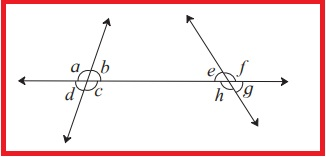# Maharashtra Board Class 8 Math Parallel lines and transversal Chapter Practice Set 1 Solution

Maha Board (MSBHSE) Class 8 Math (गणित) Parallel lines and transversal Practice Set 2.1 Solution (Page No. 6) in our website. Here candidates of Marathi Medium & English Medium of Maharashtra State can follow this to get Most Easy Solution of Maharashtra Board Class 8 Chapter 2: Parallel lines and transversal (Practice Set 2.1).

### Maharashtra Board Class 8 Math Practice Set 2.1 Solution | BalBharati Class 8 Mathematics Chapter 2 Solution:

 Subject Mathematics (गणित) Class 8 (८) Chapter 2 (२) Practice Set 2.1 (२. १)

### Practice Set 2.1 Solution (Page No. 6)

(1) In the adjoining figure, each angle is shown by a letter. Fill in the boxes with the  help of the figure. Corresponding angles.(1) <p and ____

(2)  <q and ____

(3)  <r and ____

(4)  <s and ____

Interior alternate angles.

(5) <s and ____

(6)   <w and ____

(2) Observe the angles shown in the  figure and write the following pair of angles.(1) Interior alternate angles

(2) Corresponding angles

(3) Interior angles

SOLUTION Number 1 & 2:Latest Edition BalBharati Math Solution for Standard 8th Chapter 2.1. Any Kinds of problem to understand, Feel free to comment us below. Thank You.

Updated: February 12, 2020 — 11:48 am